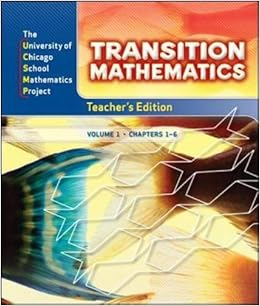• 8th Grade -- Algebra and Integrated Concepts

Students will build on concepts developed in pre algebra.  The pace of the course is designed to challenge the students. Students will gain a strong foundation in both algebra and geometry concepts, with an emphasis on deep understanding and applications to problem solving.

Concepts include: uses of variables,  linear sentences, slopes and lines, exponents and powers, graphing, quadratic equations and square roots, polynomials, related concepts of geometry, and problem solving.

Text: UCSMP AlgebraStudents will review pre-algebra concepts developed in the 6th grade curriculum, The pace of the course is designed to challenge the students. Students will extend their understanding of mathematical concepts to the realm of algebraic thinking. Concepts include:

logical reasoning, geometry concepts,iIntegers,) using and applying fractions, decimals, and percents, inequalities, proportions and proportional thinking, problem solving strategies, and uses of variables and operations in algebra.

Text: UCSMP TransitionNotes for Parents

Class Expectations